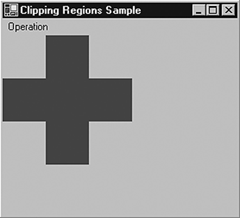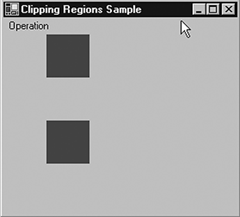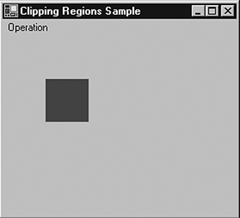# Clipping Regions Example

Listing 6.14 uses Xor to clip regions.

Listing 6.14 Using the Xor method

```Pen pen = new Pen(Color.Red, 5);
SolidBrush brush = new SolidBrush(Color.Red);
Rectangle rect1 = new Rectangle(50, 0, 50, 150);
Rectangle rect2 = new Rectangle(0, 50, 150, 50);
Region region = new Region(rect1);
region.Xor(rect2);
g.FillRegion(brush, region);
```

Figure 6.14 shows the output from Listing 6.14.

Figure 6.14. Result of the Xor methodNow if we replace Xor with Union:

```
```
```region.Union(rect2);
```
```
```

the new output looks like Figure 6.15.

Figure 6.15. Result of the Union methodNow let's replace Union with Exclude:

```
```
```region.Exclude(rect2);
```
```
```

The output looks like Figure 6.16.

Figure 6.16. Result of the Exclude methodIf we use the Intersect method:

```
```
```region.Intersect(rect2);
```
```
```

the output looks like Figure 6.17.

Figure 6.17. Result of the Intersect methodGDI+ Programming with C#
ISBN: 073561265X
EAN: N/A
Year: 2003
Pages: 145